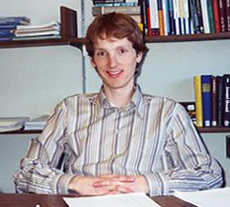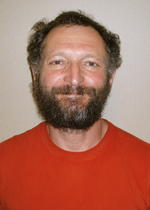Entropy and the Quantum - Tucson, Arizona, March 16-20, 2009
 main | description | program | participants | information | pictures

 The development of functional analysis, operator algebras, and related topics owes much to the field of quantum mechanics. It has provided both relevant questions and interesting concepts. Speci cally, energy and entropy estimates are routinely used in the study of partial differential equations. Moreover, the use of analytic inequalities has been perfected into an art which has generated many deep and insightful results. The goal of this school is to introduce these topics to young mathematicians; namely those at the PhD or postdoctoral level. The school will start with an introduction to quantum mechanics by Jan Wehr that will review the basic concepts from a mathematical perspective. The main part of the school will consist of three lectures given by Eric Carlen, Bruno Nachtergaele, and Robert Seiringer, all experts in their fields.Eric Carlen Trace Inequalities and Quantum Entropy A state rho in quantum statistical mechanics is given by a density matrix, i.e. a positive definite symmetric operator with Tr rho = 1. The entropy S(rho) is then given by S(rho) = -Tr rho ln rho, and the relative entropy between two states rho and mu, is S(rho,mu) = Tr[rho ln rho - rho ln mu]. These quantities play an essential role in quantum statistical mechanics. For example, they are used to express variational principles which characterize equilibrium states, to describe correlations between different systems, and to illustrate properties of systems interacting with heat baths. In these types of applications, the model often consists of many interacting subsystems. Inequalities such as the strong subadditivity of quantum entropy, originally due to Lieb and Ruskai, that governs how the entropy of the state of the whole system relates to the entropy of the state of various subsystems, are of fundamental importance. Since then, other trace inequalities allowing one to gain control over observables pertaining to the whole system in terms of simpler observables have continued to be developed and applied. What has resulted from this study is a rich body of inequalities and convexity results applicable to a wide range of functionals relevant in quantum statistical mechanics. All the same, there remain a number of open questions that are easy to state and understand, but whose proof would likely lead to a better understanding of the field in general. This course will give an introduction, at a level appropriate for beginning graduate students, to the theory of convex trace functions. Classical results, such as Lieb's proof of the convexity of the Wigner-Yanasi skew information rho mapsto -Tr([rho^{1/2},H])^2, will be discussed in detail as well as a variety of other results pertaining to more recent research. With applications to quantum computing and information, this topic of current interest is surprisingly accessible. The course will continue with some applications in quantum statistical mechanics, both equilibrium and non-equilibrium, and an introduction to open problems.Bruno Nachtergaele Quantum Entropy in Condensed Matter and Information Theory Condensed matter physics is primarily concerned with low-temperature properties of strongly interacting systems of spins and electrons. This means that the focus is on ground state properties and the nature of the low-lying excitations of the model Hamiltonians describing the systems of interest. Quantum information and compuation have added attention to this subject with specific questions about models with properties deemed to be suitable for the implemetation of quantum computation devices. For fundamental reasons, quantum systems, unlike classical ones, generically fluctuate at zero temperature. It is therefore not a surprise that entropy is a very useful tool to study ground states of quantum systems. In the context of quantum information theory, quantum entropy has been used as an effective tool to quantify entanglement, a notion describing the essential "quantumness" of the state and that is directly related to its usefulness for quantum computation. This course will introduce the main mathematical techniques that are at the basis of several interesting developments in this area. Some are new, such as the dynamical equations introduced by Hastings (which he called quasi-adiabatic evolution), and others have recently been refined and extended, such as Lieb-Robinson bounds and matrix product states, a.k.a. finitely correlated states. Several applications of these techniques will be discussed in detail, such as theorems connecting ground state properties and aspects of the low-lying spectrum, and partial results about the area law conjecture for the local entropy of quantum ground states. Due attention will be given to the discussion of important open problems for future research. Note added during the course: A \$50 price has been promised for the first proof of the area law in dimension greater than 1!Robert Seiringer Inequalities for Schroedinger Operators and Applications Schroedinger operators are of central importance in quantum mechanics. They constitute a subfield of partial differential equations and have many applications besides quantum mechanics, for instance in the study of turbulence and other nonlinear phenomena. A key to understanding stability of quantum mechanical systems is the uncertainty principle, which in mathematical terms is expressed in terms of Hardy and Sobolev inequalities for L^p norms of gradients of functions on R^d. For large quantum systems, stability is much more subtle and can be understood only via more refined types of uncertainty principles, which are known as Lieb-Thirring inequalities. These are bounds on sums of powers of the eigenvalues of Schroedinger operators, which become relevant due to antisymmetry of many-particle wavefunctions, which is also known as the Pauli principle. Recent research has focused on further understanding the relation between Hardy and Sobolev inequalities on the one hand, and Lieb-Thirring inequalities on the other hand. It turns out that the usual Lieb-Thirring inequalities can be improved by incorporating the Hardy term, and also the Hardy inequality can be improved by a (subcritical) Sobolev term. In fact, these two improvements are intimately related. The resulting inequalities have recently been applied successfully to a long-standing problem in quantum mechanics, concerning the stability of matter when both effects of special relativity and the interaction with electromagnetic fields are taken into account. Further applications may be found in other areas, such as quantum chemistry or even astronomy with stability of stars. The purpose of this course is to explain the connections between Hardy, Sobolev and Lieb-Thirring inequalities. Moreover, their usefulness will be demonstrated in several applications in analysis and mathematical physics.Jan Wehr Quantum Physics from Zero Many young mathematicians have attended a basic undergraduate course on quantum mechanics as is usually taught by a physicist. Such a class is typically insufficient to understand the necessary background and critical issues which will be at the heart of this school. Since our goal is to reach a broad audience, we will provide this introductory mini-course to present and develop the main ideas in a clear and concise form. Relevant topics include spaces of functions, the Schroedinger equation, positive linear functionals (the states in quantum mechanics), the unitary group of evolution, and a few concrete models. A special emphasis will be given to those topics discussed within the other courses of the school and specific themes which will arise in the lectures on current research projects.
 main | description | program | participants | information | pictures